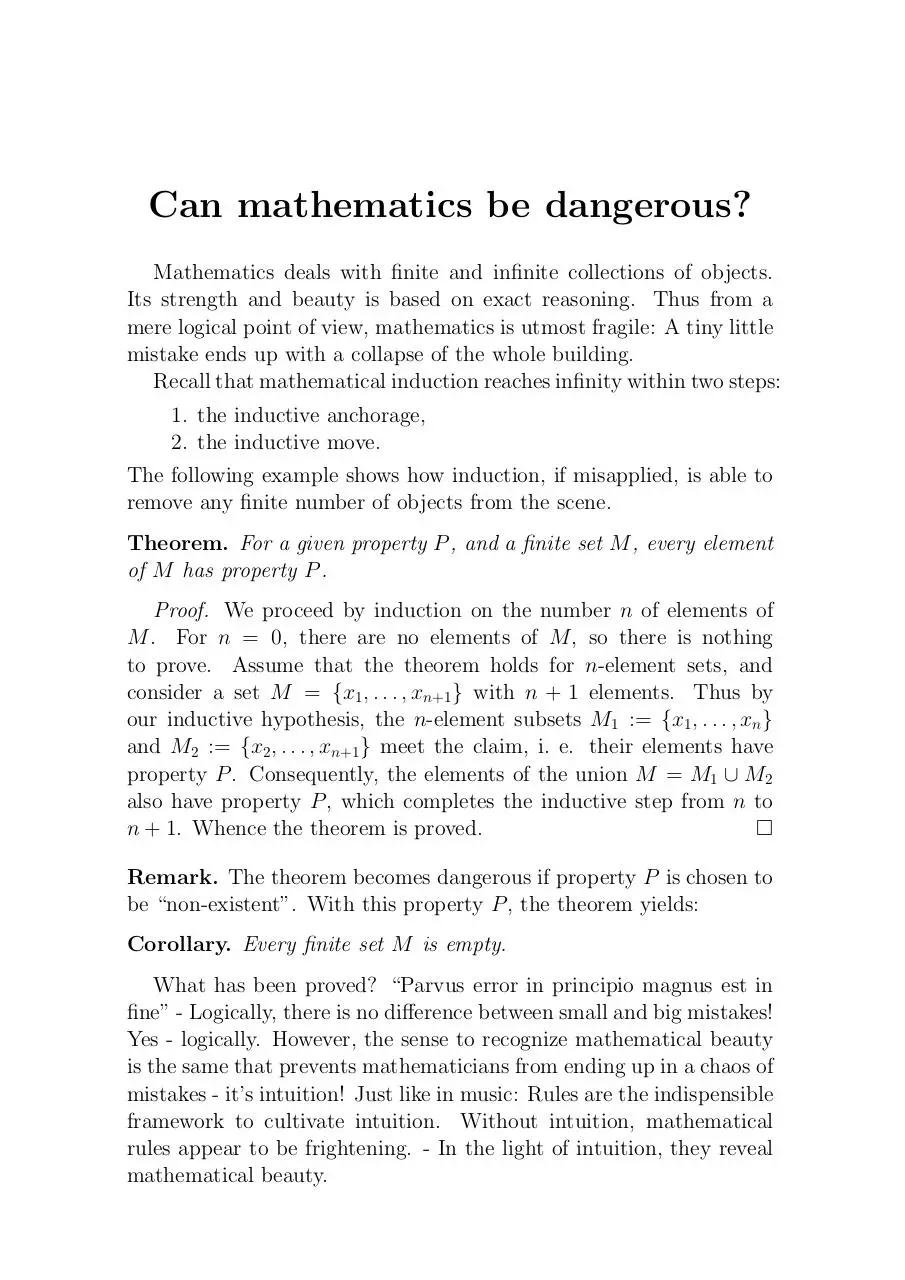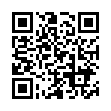# ind1 (PDF)

### File information

This PDF 1.3 document has been generated by / ESP Ghostscript 815.03, and has been sent on pdf-archive.com on 08/11/2015 at 16:34, from IP address 95.112.x.x. The current document download page has been viewed 351 times.
File size: 24.9 KB (1 page).
Privacy: public file### File preview

Can mathematics be dangerous?
Mathematics deals with finite and infinite collections of objects.
Its strength and beauty is based on exact reasoning. Thus from a
mere logical point of view, mathematics is utmost fragile: A tiny little
mistake ends up with a collapse of the whole building.
Recall that mathematical induction reaches infinity within two steps:
1. the inductive anchorage,
2. the inductive move.
The following example shows how induction, if misapplied, is able to
remove any finite number of objects from the scene.
Theorem. For a given property P , and a finite set M , every element
of M has property P .
Proof. We proceed by induction on the number n of elements of
M . For n = 0, there are no elements of M , so there is nothing
to prove. Assume that the theorem holds for n-element sets, and
consider a set M = {x1 , . . . , xn+1 } with n + 1 elements. Thus by
our inductive hypothesis, the n-element subsets M1 := {x1 , . . . , xn }
and M2 := {x2 , . . . , xn+1 } meet the claim, i. e. their elements have
property P . Consequently, the elements of the union M = M1 ∪ M2
also have property P , which completes the inductive step from n to
n + 1. Whence the theorem is proved.

Remark. The theorem becomes dangerous if property P is chosen to
be “non-existent”. With this property P , the theorem yields:
Corollary. Every finite set M is empty.
What has been proved? “Parvus error in principio magnus est in
fine” - Logically, there is no difference between small and big mistakes!
Yes - logically. However, the sense to recognize mathematical beauty
is the same that prevents mathematicians from ending up in a chaos of
mistakes - it’s intuition! Just like in music: Rules are the indispensible
framework to cultivate intuition. Without intuition, mathematical
rules appear to be frightening. - In the light of intuition, they reveal
mathematical beauty.

ind1.pdf (PDF, 24.9 KB)

#### HTML Code

Copy the following HTML code to share your document on a Website or Blog